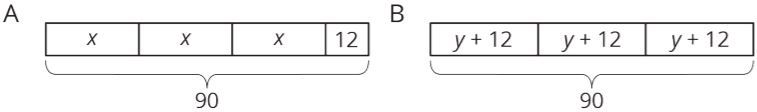PSABM6NC - Student Facing Task---Acc7.3 Lesson 6 Distinguishing between Two Types of Situations (7.EE.B.3, 7.EE.B.4.a)

Which equation doesn't belong? Explain.

4(x + 3) = 9

4 + 3x = 9

4 * x + 12 = 9

9 = 12 + 4x

Below is a set of equations. Sort the equations into 2 categories of your choosing. Then, sort the equations into 2 categories in a different way. Be prepared to explain the meaning of your categories.

100 = 8(x + 9)

9(x + 7) = 100

8(9 + x) = 100

(x + 7) * 9 = 100

100 = (9 + x) * 8

100 = 8x + 72

9x + 63 = 100

72 + 8x = 100

9x + 63 = 100

100 = 72 + 8x

Part A)Story 1: Lin had 90 flyers to hang up around the school. She gave 12 flyers to each of three volunteers. Then she took the remaining flyers and divided them up equally between the three volunteers.

Story 2: Lin had 90 flyers to hang up around the school. After giving the same number of flyers to each of three volunteers, she had 12 left to hang up by herself.

Which diagram represents story 1?

Select one:
Part B)

In each diagram, what part of the story does the variable represent?

Part C)

Write an equation corresponding to each story. If you get stuck, use the diagram.

Part D)

What is the value of the variable in story 1?

y = _____

Type your answer below as a number (example: 5, 3.1, 4 1/2, or 3/2):
Part E)

What is the value of the variable in story 2?

x = _____

Type your answer below as a number (example: 5, 3.1, 4 1/2, or 3/2):
Part A)

A tutor is starting a business. In the first year, they start with 5 clients and charge \$10 per week for an hour of tutoring with each client. For each year following, they double the number of clients and the number of hours each week. Each new client will be charged 150% of the charges of the clients from the previous year.

Organize the weekly earnings for each year in a table. Draw your table on a sheet of paper and upload your table using the image icon.

Part B)

A tutor is starting a business. In the first year, they start with 5 clients and charge \$10 per week for an hour of tutoring with each client. For each year following, they double the number of clients and the number of hours each week. Each new client will be charged 150% of the charges of the clients from the previous year.

Assuming a full-time week is 40 hours per week, how many years will it take to reach full time?

______ years

Type your answer below as a number (example: 5, 3.1, 4 1/2, or 3/2):
Part C)

A tutor is starting a business. In the first year, they start with 5 clients and charge \$10 per week for an hour of tutoring with each client. For each year following, they double the number of clients and the number of hours each week. Each new client will be charged 150% of the charges of the clients from the previous year.

Assuming a full-time week is 40 hours per week, how many years will it take to reach full time and how many new clients will be taken on that year?

______ clients

Type your answer below as a number (example: 5, 3.1, 4 1/2, or 3/2):
Part D)

A tutor is starting a business. In the first year, they start with 5 clients and charge \$10 per week for an hour of tutoring with each client. For each year following, they double the number of clients and the number of hours each week. Each new client will be charged 150% of the charges of the clients from the previous year.

After reaching full time, what is the tutor’s annual salary if they take 2 weeks of vacation? (Don't use \$ in your answer)